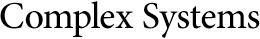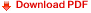## Exponential Period of Neuronal Recurrence Automata with Excitatory MemoryRené Ndoundam
The sequences generated by a neuronal recurrence equation with memory of the form $x ⁡ ( n ) = 1 [ ∑ i = 1 h a i ⁢ x ⁡ ( n - i ) - θ ]$, where h is the size of the memory, are studied. It is shown that in the case where all the parameters $( a i ) 1 ≤ i ≤ h$ are positive reals, there exists a neuronal recurrence equation of memory length h that generates a sequence of period $Ω ⁡ ( e h ⁡ ( ln ⁡ ( h ) ) 2 3 )$. This result shows that in the case where all the weighting coefficients are positive reals, the neuronal recurrence equation exhibits a complex behavior.